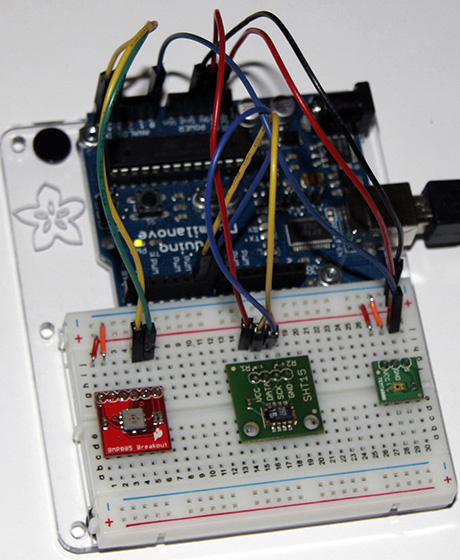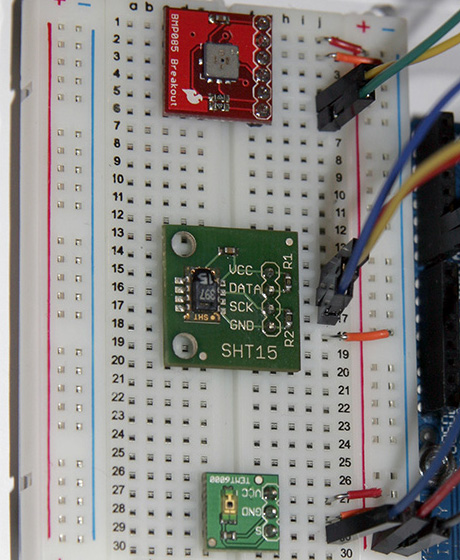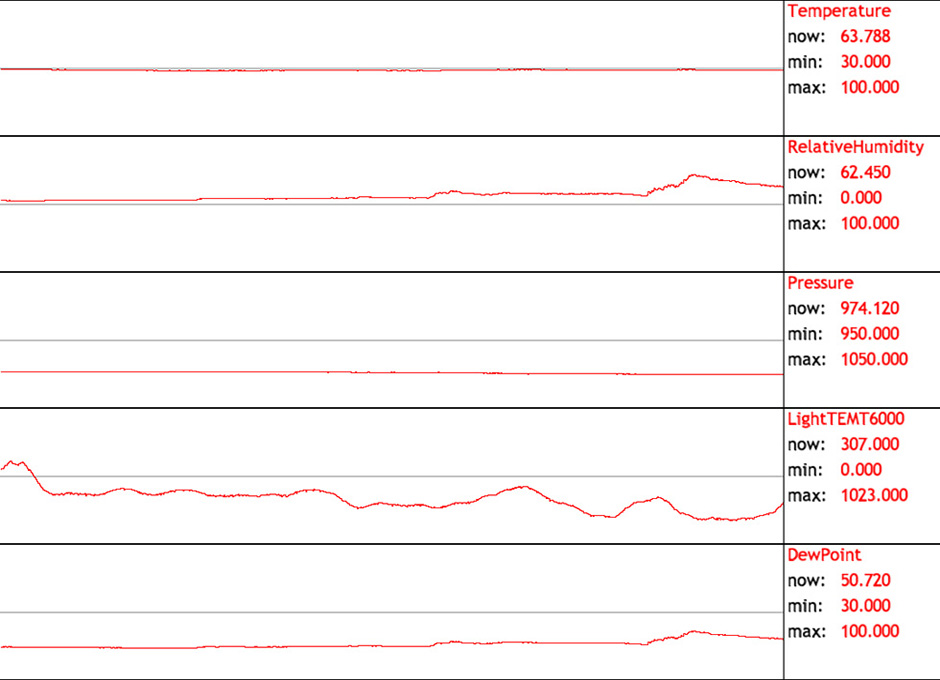The goal of this project is to log some weather data and be able to access it from anywhere. There is some sensor data (temperature, relative humidity, pressure, and ambient light) and some computed data (dew point). You can see a few reports at my sensors site:

All source code is available from my local download or from github.com/larsi-org/WeatherStation

Like most logging projects this is almost like lego - you choose the sensors you like, connect them to the pins of a microcontroller that the sensor protocol require, and copy and paste all the code that is needed to talk to the individual sensors. I wanted to keep it simple, which means this project requires a host computer that collects the data and sends it out. The host computer can be replaced by an Arduino Ethernet shield or some 802.11 wireless connection, but this would make it slightly more complicated and I would loose the local graphing.

The microcontroller is responsible to setup all the pins to the correct mode (input/output) and to initialize all sensors. The main loop will just read the current value of all sensors and send it out over the serial port. This was relatively easy for me, because I already owned all the sensors and I had figured out the communication before I started this project. Here are the links to the subproject:

The sensors are connected as follows:

Sensor Sensor Pin Arduino Pin
SHT15 GND GND
SCK DIGITAL 7
DATA DIGITAL 6
VCC 5V
BMP085 VCC 3V3
GND GND
EOC
XCLR
SCL ANALOG 5
SDA ANALOG 4
TEMT6000 VCC 5V
GND GND
S ANALOG 0

The software has a few independent pieces and each is not very complicated. If a logging service is used, like www.thingspeak.com, only three pieces are necessary:

• an Arduino program runs on a microcontroller (Arduino) and talks to all the sensors and reports to the host computer over the serial port
• a Processing program runs on the host computer and collects the data from the serial port, graphs each sensor output locally, and sends it to a web logger with an HTML request
• a web page calls the different graphs and displays them

If you want to set up your own web logging than you need a few more additional pieces:

• a PHP web logger runs on a web server and stores the data in a remote MySQL database
• a PHP web graph program runs on a web server and queries the remote MySQL database and calls Google Chart Tools to generate the graphs

## Arduino

The Arduino code is just copied together from my previous projects:

```/*
* WeatherStation by Lars Schumann (make.larsi.org)
*
* Uses the following senors: SHT15, BMP085, and TEMT6000
*/

#include "Wire.h"

#define PIN_SDA  6
#define PIN_SCL  7

const unsigned char oversampling_setting = 3; //oversamplig for measurement
const unsigned char pressure_waittime = { 5, 8, 14, 26 };

//just taken from the BMP085 datasheet
int ac1;
int ac2;
int ac3;
unsigned int ac4;
unsigned int ac5;
unsigned int ac6;
int b1;
int b2;
int mb;
int mc;
int md;

void bmp085_read_temperature_and_pressure(int* temperature, long* pressure) {
long x1, x2, x3, b3, b5, b6, p;
unsigned long b4, b7;

//calculate the temperature
x1 = ((long)ut - ac6) * ac5 >> 15;
x2 = ((long) mc << 11) / (x1 + md);
b5 = x1 + x2;
*temperature = (b5 + 8) >> 4;

//calculate the pressure
b6 = b5 - 4000;
x1 = (b2 * (b6 * b6 >> 12)) >> 11;
x2 = ac2 * b6 >> 11;
x3 = x1 + x2;

//b3 = (((int32_t) ac1 * 4 + x3)<> 2;

if (oversampling_setting == 3) b3 = ((int32_t) ac1 * 4 + x3 + 2) << 1;
if (oversampling_setting == 2) b3 = ((int32_t) ac1 * 4 + x3 + 2);
if (oversampling_setting == 1) b3 = ((int32_t) ac1 * 4 + x3 + 2) >> 1;
if (oversampling_setting == 0) b3 = ((int32_t) ac1 * 4 + x3 + 2) >> 2;

x1 = ac3 * b6 >> 13;
x2 = (b1 * (b6 * b6 >> 12)) >> 16;
x3 = ((x1 + x2) + 2) >> 2;
b4 = (ac4 * (uint32_t) (x3 + 32768)) >> 15;
b7 = ((uint32_t) up - b3) * (50000 >> oversampling_setting);
p = b7 < 0x80000000 ? (b7 * 2) / b4 : (b7 / b4) * 2;

x1 = (p >> 8) * (p >> 8);
x1 = (x1 * 3038) >> 16;
x2 = (-7357 * p) >> 16;
*pressure = p + ((x1 + x2 + 3791) >> 4);
}

write_register(0xf4,0x2e);
delay(5); //longer than 4.5 ms
}

void bmp085_get_cal_data() {
//Serial.print("AC1: ");
//Serial.println(ac1,DEC);
//Serial.print("AC2: ");
//Serial.println(ac2,DEC);
//Serial.print("AC3: ");
//Serial.println(ac3,DEC);
//Serial.print("AC4: ");
//Serial.println(ac4,DEC);
//Serial.print("AC5: ");
//Serial.println(ac5,DEC);
//Serial.print("AC6: ");
//Serial.println(ac6,DEC);
//Serial.print("B1: ");
//Serial.println(b1,DEC);
//Serial.print("B2: ");
//Serial.println(b1,DEC);
//Serial.print("MB: ");
//Serial.println(mb,DEC);
//Serial.print("MC: ");
//Serial.println(mc,DEC);
//Serial.print("MD: ");
//Serial.println(md,DEC);
}

write_register(0xf4,0x34+(oversampling_setting<<6));
delay(pressure_waittime[oversampling_setting]);

unsigned char msb, lsb, xlsb;
Wire.endTransmission();

while(!Wire.available()) {
// waiting
}
while(!Wire.available()) {
// waiting
}
while(!Wire.available()) {
// waiting
}
return (((long)msb<<16) | ((long)lsb<<8) | ((long)xlsb)) >>(8-oversampling_setting);
}

void write_register(unsigned char r, unsigned char v)
{
Wire.send(r);
Wire.send(v);
Wire.endTransmission();
}

{
unsigned char v;
Wire.endTransmission();

while(!Wire.available()) {
// waiting
}
return v;
}

{
unsigned char msb, lsb;
Wire.endTransmission();

while(!Wire.available()) {
// waiting
}
while(!Wire.available()) {
// waiting
}
return (((int)msb<<8) | ((int)lsb));
}

void resetSHT()
{
pinMode(PIN_SDA, OUTPUT);
pinMode(PIN_SCL,  OUTPUT);

shiftOut(PIN_SDA, PIN_SCL, LSBFIRST, 255);
shiftOut(PIN_SDA, PIN_SCL, LSBFIRST, 255);

digitalWrite(PIN_SDA, HIGH);
for(int i = 0; i < 15; i++) {
digitalWrite(PIN_SCL, LOW);
digitalWrite(PIN_SCL, HIGH);
}
}

//Specific SHT start command
void startSHT()
{
pinMode(PIN_SCL,  OUTPUT);
pinMode(PIN_SDA, OUTPUT);
digitalWrite(PIN_SDA, HIGH);
digitalWrite(PIN_SCL,  HIGH);
digitalWrite(PIN_SDA, LOW);
digitalWrite(PIN_SCL,  LOW);
digitalWrite(PIN_SCL,  HIGH);
digitalWrite(PIN_SDA, HIGH);
digitalWrite(PIN_SCL,  LOW);
}

void writeByteSHT(byte data)
{
pinMode(PIN_SCL,  OUTPUT);
pinMode(PIN_SDA, OUTPUT);

//  digitalWrite(PIN_SDA,LOW);
shiftOut(PIN_SDA,PIN_SCL, MSBFIRST, data);

pinMode(PIN_SDA, INPUT);

//Wait for SHT15 to acknowledge by pulling line low

digitalWrite(PIN_SCL, HIGH);
digitalWrite(PIN_SCL, LOW);  //Falling edge of 9th clock

//wait for SHT to release line

//wait for SHT to pull data line low to signal measurement completion
//This can take up to 210ms for 14 bit measurments
int i = 0;
i += 10;
if (i >= 1000) break;
delay(10);
}

//debug
//Serial.print("Response time = ");
//Serial.println(i);
}

//Read 16 bits from the SHT sensor
{
int cwt = 0;

pinMode(PIN_SDA, INPUT);
pinMode(PIN_SCL,  OUTPUT);

digitalWrite(PIN_SCL, LOW);

for(int i = 0; i < 17; i++) {
if(i != 8) {
digitalWrite(PIN_SCL, HIGH);
cwt = cwt << 1 | digitalRead(PIN_SDA);
digitalWrite(PIN_SCL, LOW);
}
else {
pinMode(PIN_SDA, OUTPUT);
digitalWrite(PIN_SDA, LOW);
digitalWrite(PIN_SCL,  HIGH);
digitalWrite(PIN_SCL,  LOW);
pinMode(PIN_SDA, INPUT);
}
}

//leave clock high??
digitalWrite(PIN_SCL, HIGH);

//Serial.println();
return cwt;
}

int getTempSHT()
{
startSHT();
writeByteSHT(B0000011);
}

int getHumidSHT()
{
startSHT();
writeByteSHT(B00000101);
}

void setup()
{
Serial.begin(9600); // start serial for output
//Serial.println("Setting up BMP085");

pinMode(PIN_SDA, OUTPUT);
pinMode(PIN_SCL, OUTPUT);

Wire.begin();
bmp085_get_cal_data();
resetSHT();
delay(2000);
}

void loop()
{
int temperature = 0;
long pressure = 0;

float val;

val = (float)getTempSHT();
float tempC = -40.0 + 0.01 * val;

val = (float)getHumidSHT();
float humid = -4.0 + 0.0405 * val + -0.0000028 * val * val;

// http://en.wikipedia.org/wiki/Dew_point
float a = 17.271;
float b = 237.7;
float gamma = log(humid / 100) + a / (b / tempC + 1);
float dewPoint = b / (a / gamma - 1);

Serial.print(tempC);
Serial.print(",");
//Serial.print(temperature / 10, DEC);
//Serial.print(".");
//Serial.print(temperature % 10, DEC);
//Serial.print(",");
Serial.print(humid);
Serial.print(",");
Serial.print(pressure);
Serial.print(",");
Serial.print(",");
Serial.println(dewPoint);
delay(10000);
}```

It writes to the serial port every 10 seconds all the sensor value separated by comma (temperature in °C, relative humidity in %, pressure in Pa, ambient light (0-1023), dew point in °C):

```19.53,74.03,97493,2,14.77
19.48,74.09,97503,0,14.74
19.51,74.01,97509,0,14.75
19.51,74.01,97502,1,14.75
19.53,73.98,97495,1,14.76```

## Processing

The Processing code uses a modified version of the arduinoscope's channel library:

```/*
* Channel.java by Lars Schumann (make.larsi.org)
*
* Draws a float array as a graph
*
* It is based on Channel.java by David Konsumer <david.konsumer@gmail.com>
* but had to be modified
*/

import processing.core.PApplet;
import processing.core.PConstants;

public class Channel implements PConstants
{
PApplet parent;
String label;
float minval;
float maxval;
int dimX; // width
int dimY; // height
int offY; // y start position
int COLOR_GRAPH; // color for lines
int COLOR_CENTER; // color for center line

private float[] values; // all values in the graph

public Channel(PApplet parent, String label, float minval, float maxval, int dimX, int dimY, int offY)
{
this.parent = parent;
this.label = label;
this.minval = minval;
this.maxval = maxval;
this.dimX = dimX;
this.dimY = dimY;
this.offY = offY;

// set some defaults
COLOR_GRAPH = 0xFFFF0000; // red
COLOR_CENTER = 0xFF999999; // gray

values = new float[dimX];
for (int i = 0; i < dimX; i++) values[i] = minval;
}

public void draw()
{
// draw center line
parent.stroke(COLOR_CENTER);
parent.line(0, offY + (dimY/2), dimX, offY + (dimY/2));

parent.stroke(COLOR_GRAPH);
int yOld = getY(0);
for (int x = 1; x < dimX; x++) {
int yNew = getY(x);
parent.line(x, yOld, x, yNew);
yOld = yNew;
}
}

{
for (int i = 0; i < dimX - 1; i++) values[i] = values[i + 1];
values[dimX - 1] = val;
if (val < minval) minval = val;
if (val > maxval) maxval = val;
}

public float getCurrentData()
{
return values[dimX - 1];
}

private int getY(int index)
{
return parent.round(parent.map(values[index], minval, maxval, offY + dimY - 2, offY + 1));
}
}```

The ThingSpeak.pde is the main application. It gets data from the serial port and updates the graphs. Every 5 minutes it send the data to ThingSpeak. It constructs an URL that looks like this (all values in <> need to be replaced by the real values):

```http://api.thingspeak.com/update?key=<key>&field1=<value1>&field2=<value2>&field3=<value3>&field4=<value4>&field5=<value5>
```

Processing does not have any direct way to request an URL from a server, but Processing allows to use any Java code. So, this works:

```  String url = ;
try {
while (line != null) {
System.out.println(line);
}
}
catch (java.io.IOException e) {
e.printStackTrace();
}```

The complete code is here (you have to adjust your ThingSpeak update key and the port number of your serial connection):

```/*
* ThingSpeak.pde by Lars Schumann (make.larsi.org)
*
* Collects weather data from an Arduino and
* sends the data to ThingSpeak every 5 minutes.
*/

import processing.serial.*;

// Logging server
String SERVER   = "http://api.thingspeak.com/update?key=0000000000000000";

// Station channels
String labels[] = { "Temperature", "RelativeHumidity", "Pressure", "LightTEMT6000", "DewPoint" };
String fields[] = {      "field1",           "field2",   "field3",        "field4",   "field5" };
float  mins[]   = {            30,                  0,        950,               0,         30 };
float  maxs[]   = {           100,                100,       1050,            1023,        100 };
float  m[]      = {           1.8,                  1,       0.01,               1,        1.8 };
float  n[]      = {            32,                  0,          0,               0,         32 };

Channel channels[] = new Channel[labels.length];

Serial port;

int LINE_FEED=10;

// setup vals from serial
float[] vals = new float[labels.length];
long currentID = 0;
long lastID    = 2; // skip first two values
String lastTime = "";

void setup()
{
size(1080, 800, P2D);
frameRate(1);
background(0);

// set these up under tools/create font, if they are not setup.

int dimX = width - 180; // 180 margin for text
int dimY = height / labels.length;

for (int i = 0; i < labels.length; i++)
channels[i] = new Channel(this, labels[i], mins[i], maxs[i], dimX, dimY, dimY * i);

println("Available serial ports:");
println(Serial.list());

port = new Serial(this, Serial.list(), 9600);

// clear and wait for linefeed
port.clear();
port.bufferUntil(LINE_FEED);
}

void draw()
{
background(0xFFFFFFFF); // white

// update channels
if (currentID > lastID) {
for (int i = 0; i < labels.length; i++) channels[i].addData(m[i] * vals[i] + n[i]);
lastID = currentID;
}

// al the same
int dimX = channels.dimX;
int dimY = channels.dimY;

// draw channels
for (int i = 0; i < labels.length; i++) {
int offY = channels[i].offY;

// draw lines
stroke(0xFF000000); // black
line(0, offY, width, offY);
line(0, offY + dimY - 1, width, offY + dimY - 1);

channels[i].draw();

fill(0xFFFF0000); // red
text(channels[i].label,            dimX +  5, offY +  20);
text(channels[i].getCurrentData(), dimX + 60, offY +  50);
text(channels[i].minval,           dimX + 60, offY +  80);
text(channels[i].maxval,           dimX + 60, offY + 110);
fill(0xFF000000); // black
text("now:", dimX + 5, offY + 50);
text("min:", dimX + 5, offY + 80);
text("max:", dimX + 5, offY + 110);
}

// draw text seperator, based on first scope
stroke(0xFF000000); // black
line(0,         0, 0,         height);
line(dimX,      0, dimX,      height);
line(width - 1, 0, width - 1, height);
}

// handle serial data
void serialEvent(Serial p)
{
if (data != null) {
String[] data_split = split(data, ',');
for (int i = 0; i < labels.length; i++) vals[i] = float(data_split[i]);

DateFormat dateFormat = new SimpleDateFormat("yyyy-MM-dd");
java.util.Date date = new java.util.Date();
int m = 5 * (date.getMinutes() / 5);
int h = date.getHours();
String currentTime = dateFormat.format(date) + " " + twoDigits(h) +  ":" + twoDigits(m) + ":00";
String url = SERVER;
if (!currentTime.equals(lastTime)) {
lastTime = currentTime;
for (int i = 0; i < labels.length; i++) url += "&" + fields[i] + "=" + vals[i];
try {
while (line != null) {
System.out.println(line);
}
}
catch (java.io.IOException e) {
e.printStackTrace();
}
}
}
currentID++;
}

String twoDigits(int v)
{
return "" + (v < 10 ? "0" : "") + v;
}```

This is an example of the graphical output:## Show the weather condition

ThingSpeak makes it easy to access your own data. Here is just an example, but much better graphs are possible:

```<html>
<title>Weather Condition</title>

<body>
<h1>Weather Condition <?php print(date('m/d/Y')); ?></h1>
<table width="100%">
<tr>
<td><div align="center"><iframe width="480" height="250" style="border: 1px solid #cccccc;" src="https://thingspeak.com/channels/346/charts/1?timescale=10"></iframe></div></td>
<td><div align="center"><iframe width="480" height="250" style="border: 1px solid #cccccc;" src="https://thingspeak.com/channels/346/charts/2?timescale=10"></iframe></div></td>
</tr>
<tr>
<td><div align="center"><iframe width="480" height="250" style="border: 1px solid #cccccc;" src="https://thingspeak.com/channels/346/charts/3?timescale=10"></iframe></div></td>
<td><div align="center"><iframe width="480" height="250" style="border: 1px solid #cccccc;" src="https://thingspeak.com/channels/346/charts/4?timescale=10"></iframe></div></td>
</tr>
<tr>
<td><div align="center"><iframe width="480" height="250" style="border: 1px solid #cccccc;" src="https://thingspeak.com/channels/346/charts/5?timescale=10"></iframe></div></td>
<td>&nbsp;</td>
</tr>
</table>
</body>
</html>```

## Web Logger (PHP, MySQL)

This is the more advanced section, if you want to set up your own web logging service and use Google's Chart API to visualize the graphs.

```CREATE TABLE `datalogger` (
`DateTime` datetime NOT NULL,
`Location` enum('Attic','BathRoom2','Bathroom3','Bedroom2','Bedroom2','Bench','Den','DiningRoom','FamilyRoom','Garage','Garden','GuestRoom','HisOffice','HerOffice','KidsRoom1','KidsRoom2','KidsRoom3','Kitchen','LivingRoom','ManCave','MasterBathRoom','MasterBedRoom','MediaRoom','Office','Porch','Study','UtilityRoom','WorkoutRoom') NOT NULL,
`Type` enum('DewPoint','Energy','LightTEMT6000','Power','Pressure','RelativeHumidity','RPM','Temperature') NOT NULL,
`Value` float NOT NULL
) ENGINE=MyISAM DEFAULT CHARSET=utf8;
```

## Web Graph (MySQL, PHP, Google's Chart API)